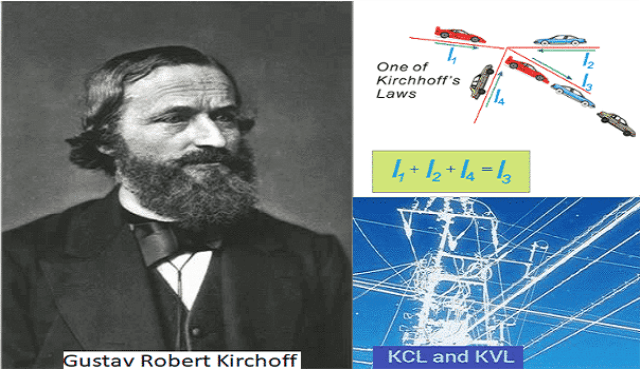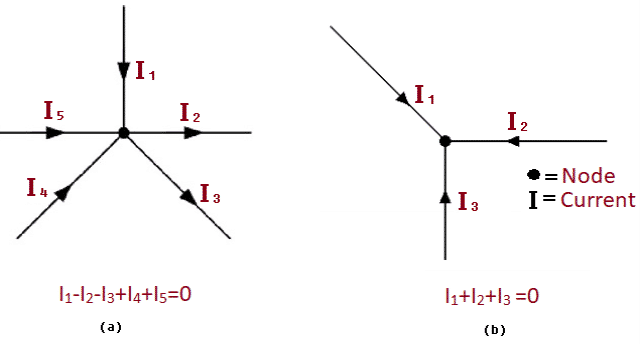Saturday, December 9, 2023
HomePower Generation & DistributionKirchhoff's LawsKirchhoff's Laws of Current & Voltage - Application, Advantage, Limitation

# Kirchhoff’s Laws of Current & Voltage – Application, Advantage, Limitation

Kirchhoff’s Laws act as the best tool for circuit analysis techniques. Georg Ohm’s work (Ohm’s law) formed the foundation to create Kirchhoff’s Current Law (KCL) and Kirchhoff’s Voltage Law (KVL) by Gustav Robert Kirchhoff in 1845. The objective of this post is to give a better understanding of Kirchhoff’s Laws of Current and Voltage, its applications, advantages and limitation.

## Kirchhoff’s Laws

The laws of Kirchhoff are the two equations that address conservation of energy and charge with reference to electrical circuits. They are very important in the analysis of closed and complex electrical circuits such as bridge or T networks in which calculating voltages or currents circulating within the circuit becomes difficult using Ohm’s law alone.Fig. 1 – Introduction to Kirchhoff’s First and Second Laws

## Kirchhoff’s Laws of Current and Voltage

Kirchhoff’s Laws of Current and Voltage may be divided in two separate laws, i.e.:

• Kirchhoff’s Current Law (KCL) or First Law
• Kirchhoff’s Voltage Law (KVL) or Second Law

### Kirchhoff’s Current Law (KCL) or First Law:

The first law of Kirchhoff’s laws is Kirchhoff’s Current Law. It states that “The total current or charge entering a junction or node is exactly equal to the current leaving the node, as no charge is lost within the node“. It can also be stated as the sum of currents in a network of conductors meeting at a node is equal to zero.Fig. 2 – Visual Representation of Kirchhoff’s Current Law

From the above figure,we can infer that the sum of the currents entering and leaving a node is equal to Zero (0). The current flowing towards the junction is considered positive and the current flowing away from the node or junction is considered negative.

In other words, KCL can be defined as the algebraic sum of all the currents entering and leaving a node must be equal to zero, i.e. Iin + Iout = 0.

### Kirchhoff’s Voltage Law (KVL) or Second Law:

The second law of Kirchhoff’s laws is Kirchhoff’s Voltage Law. It states that in any closed loop network, the sum of the emf values in any closed loop is equal to the sum of the potential drops in that loop.Fig. 3 – Visual Representation of Kirchhoff’s Voltage Law

In another words, it can also be said as “The total voltage around the loop is equal to the sum of all the voltage drops within the same loop”, which is equal to zero.

## Applications of Kirchhoff’s Laws

The applications include:

• They can be used to analyze any electrical circuit.
• Computation of current and voltage of complex circuits.

• Calculation of unknown currents and voltages is easy.
• Simplification and analysis of complex closed loop circuits becomes manageable.

## Limitations of Kirchhoff’s Laws

The limitation of Kirchhoff’s both laws is that it works under the assumption that there is no fluctuating magnetic field in the closed loop. Electric fields and emf could be induced which causes the Kirchhoff’s loop rule to break in presence of a variable magnetic field.

`Also Read:Series Circuit – Working Principle, Characteristics, Applications, AdvantageWhat is Parallel Circuit – How to Make, Characteristics, ApplicationsVoltmeter – Working Principle, Voltage Sensitivity, Types and Applications`Laxmi Ashrit
Laxmi is a B.E (Electronics & Communication) and has work experience in RelQ Software as Test Engineer and HP as Technical support executive. She is an author, editor and partner at Electricalfundablog.
RELATED ARTICLES